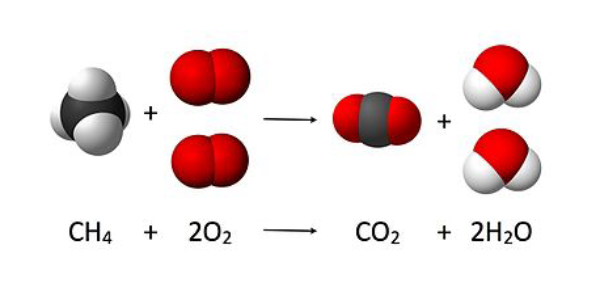What mass of NO2 is formed when NO reacts with 386 g of O2?   - ProProfs DiscussTopicsMoreProductsMore+ Ask Question

# What mass of NO2 is formed when NO reacts with 386 g of O2?  Reference: Nitrogen combines with oxygen in the atmosphere during lightning flashes to form nitrogen monoxide, NO, which then reacts further with O2 to produce nitrogen dioxide, NO2, according to the following equation:N2 (g) + O2 (g) --> 2 NO (g)2 NO (g) + O2 (g) --> 2 NO2 (g)Change Image    Delete

A. 1110 g NO2
B. 1109.99 g NO2
C. 1110.0 g NO2
D. 0.0111 g NO2

This question is part of Stoichiometry QUIZ
Asked by Bethany.herman, Last updated: Jul 29, 2020

###Request 0FollowShareAnswer AnonymouslyAnswer LaterCopy LinkC. Perez, Writer, Writer, Cleveland

The correct answer to this question is 1110 g NO2. To solve this question, one must first create an equation. Each side of the equation must have the same number of the atom's moles. This will allow the equation to be balanced.

From there, we must find the mass of the raw materials, as well as the mass of products that are formed. This can be found by multiplying the weight of each molecule by the number of moles there are. Then, to see the final answer, we can use the unitary method. This method will give us the number 1109.99125. Since that answer isn't one of the choices, we can round up to get 1110g.N. Kingsley, Writer, Columbus

When you need to figure out the weight of a special product, you must first construct an equation. The equation must be balanced with an equal number of moles of each atom on either side of the equation. You can then find the molecular weight of each molecule and multiply it by the number of moles to get the accurate mass of each raw material used and the mass of products formed.

Once you have that you can use the unitary method to find what mass will form had the starting reactants be an amount similar to the question. This will give an answer of 1110g of NO2.John Smith1110 g NO2

Given: 386 g O2 Unknown: ? g NO2 386 g O2 x (1/32.00g/mol O2) x (2 mol NO2/1 mol O2) x (46.01 g/mol NO2) = 1109.99125 = 1110 g NO2 Although 1109.99125 g NO2 is technically correct, your answer must include the correct number of significant figures (which in this instance is 3). The answer must be rounded to 1110.Search for Google imagesSelect a recommended image
Upload from your computerCancelSearch for Google imagesSelect a recommended image
Upload from your computerCancelSearch for Google imagesSelect a recommended image
Upload from your computerCancel